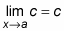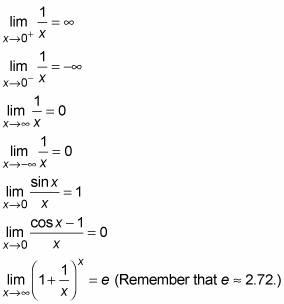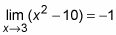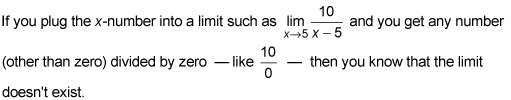##### Trigonometry Workbook For DummiesThere are two types of easy limit problems: the ones you should just memorize and the ones where you can plug in the x-number and get the answer in one step.

## Limits to memorize

You should memorize the following limits to avoid wasting time trying to figure them out. (Pay special attention to the last three limits.)(y = c is a horizontal line, so the limit — which is the function height — must equal c, regardless of the x-number.)## Plugging and chugging

You can use the plug-and-chug method to solve some limit problems. Just plug the x-number into the limit function, and if the computation results in a number, that’s your answer (don’t forget that zero divided by anything other than zero equals zero which is, of course, a number). For exampleThis method always works for limits that involve continuous functions and functions that are continuous over their entire domains. These are well-duh limit problems, and, to be perfectly frank, there is really no point to them. The limit is simply the function value.

The plug-and-chug method also works for any other type of function, including piecewise functions, unless there is a discontinuity at the x-number you plug in. (A piecewise function has separate pieces, where each part of the piecewise function has its own equation.)